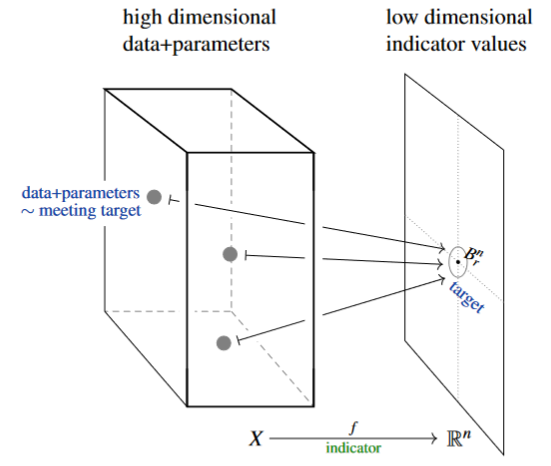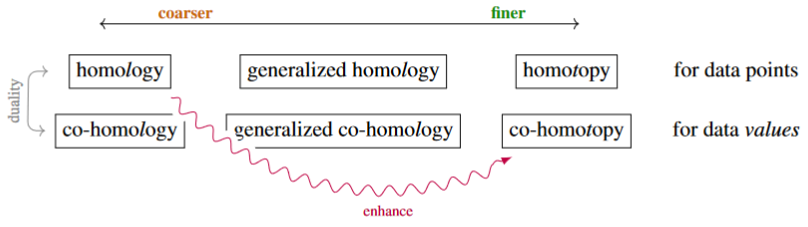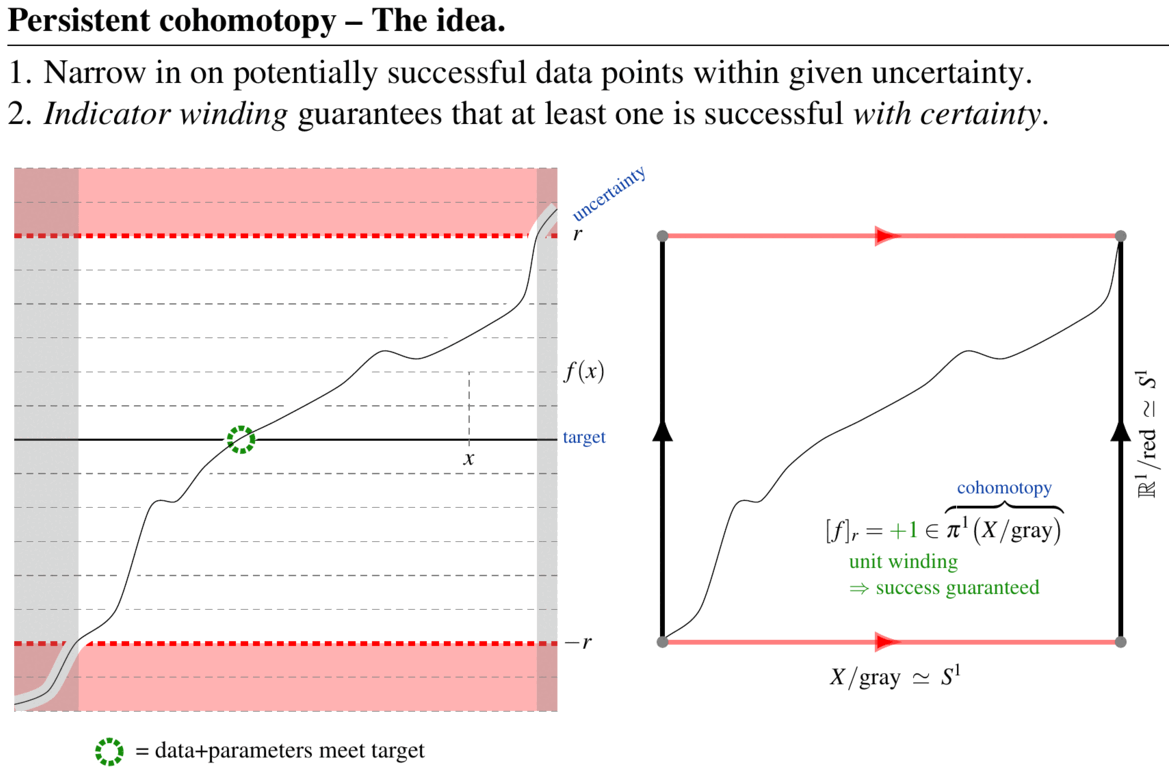# nLab persistent cohomotopy

Contents

### Context

#### Spheres

n-sphere

low dimensional n-spheres

# Contents

## Idea

### General

Persistent cohomotopy (not yet an established term) would be the study of cohomotopy in situations where the domain space and/or its map to an n-sphere may depend on a parameter, to yield not just a single cohomotopy set, but a filtered set (a filtered group when the dimension of the domain space is large enough). One concrete implementation of this general notion is considered in Franek & Krčál 2017, see below.

Generally, one may understand persistent cohomotopy both as the dual of persistent homotopy as well as a non-abelian cohomology-version of the more widely considered notion of persistent homology:

coarseintermediatefine
homologyordinary homologygeneralized homologyhomotopy
cohomologyordinary cohomologygeneralized cohomologycohomotopy
persistent homologypersistent ordinary homologypersistent generalized homologypersistent homotopy
persistent cohomologypersistent ordinary cohomologypersistent generalized cohomologypersistent cohomotopy

### Detecting data meeting targets

While persistent homology, as a tool in topological data analysis, is traditionally meant to discover persistent cycles in a given data set, it is typically unclear what a persistent cycle actually means for the practical interpretation of the data – certainly this information is not provided by the mathematics.In contrast, persistent cohomotopy in TDA is the effective answer to a concrete and common question in data analysis:

Given a large-dimensional space of data, and a small number $n$ of (real) indicator values assigned to each data point with given precision $1/r$, does any data meet a prescribed target indication precisely?

A fundamental theorem of persistent Cohomotopy (Franek, Krčál & Wagner 2018, Franek & Krčál 2017, p. 5, see Thm. below) shows that (1.) the answer to this question is detected by a certain Cohomotopy-class and (2.) in a fair range of dimensions, this Cohomotopy class is provably computable, hence the above question is effectively decidable. $\phantom{----}$ (figure from SS22)

Alternatively, with tools from persistent homology theory an answer to this question is given by the method of well groups – but (1.) it is known that well groups are in general too coarse to provide a complete answer and (2.) despite effort it remains unknown if well groups are actually computable in relevant cases, see Franek & Krčál 2016.

In this concrete sense (and generally by the above discussion), persistent cohomotopy may be understood as an enhancement or refinement of (well groups in) persistent homology:(graphics from SS 22)

### Use of cobordism theory

If the topological space $X$ of data may be assumed to be a smooth manifold (indeed, in typical examples $X$ is itself a large-dimensional Cartesian space) then persistent cohomotopy may be understood dually via Pontryagin's theorem as characterizing iso-hypersurfaces of data (close to a given target indicator) by framed cobordism theory (Franek & Krčál 2017, p. 8-9). The full implications of this relation for topological data analysis remain to be explored.

## Definition

We spell out the definition considered in Franek & Krčál 2017.

For

• $X$ a topological space,

• $n \in \mathbb{N}$ a natural number,

• $r \in \mathbb{R}_{\gt 0}$ a positive real number,

• $f \,\colon\, X \xrightarrow{\;\;\;\;\;\;} \mathbb{R}^n$ a continuous map,

such that with

• $A_r \,\coloneqq\, f^{-1}\big( \mathbb{R}^n \setminus B_r \big) \;\xhookrightarrow{i_r}\; X\;$

(the preimage under $f$ of the complement of the open ball of radius $r$ around the origin in the Cartesian space)

we have that

• $(X,A_r)$ is a CW-pair of dimension $dim(X) \leq 2n - 3$,

consider the following induced exact sequence of cohomotopy-groups (where square brackets $[X,Y]$ denote the hom-set $Ho(X,Y)$ in the classical homotopy category, hence homotopy classes of continuous functions $X \to Y$):

Now define what we may call the $n$-cohomotopy of $X$ at resolution $1/r$ relative to $f$, to be (this is FK17 (2)):

(1)$\pi^n_{(f,r)} \;\coloneqq\; image(\delta_r) \;\simeq\; \Big\{ [g/A_r] \,\in\, \pi^n(X/A_r) \,\big\vert\, (X, A_r) \xrightarrow{ \exists \, g } (\mathbb{R}^n,\, \mathbb{R}^n \setminus B_r) \Big\} \,,$

hence that subgroup of the $n$-cohomotopy group of the quotient topological space $X/A_r$ whose elements may be represented by continuous maps defined on all of $X$.

Notice that the function $f$ itself canonically represents an element

(2)$[f]_r \;\in\; \pi^n_{(f,r)}(X)$

which provides a base point, making this a pointed group.

Moreover, for $r_1 \leq r_2$ we have the evident inclusion

$A_{r_2} \xhookrightarrow{\; i_{r_1, r_2} \;} A_{r_1}$

and thus a canonical comparison homomorphisms of pointed groups:

Therefore, regarding the positive real numbers ordered by $\leq$ as a poset and thus as a category, the resulting directed diagram of pointed groups

(3)$\pi^n_{(f,\bullet)} (X) \;\colon\; (\mathbb{R}, \leq) \xrightarrow{\;\;\;} Grp^{\mathbb{Z}/}$

is the cohomotopy persistence module (FK17, p. 5, rhyming on persistence module as used in persistent homology theory) of $X$ relative to $f$.

## Properties

For $r \in \mathbb{R}_{\gt 0}$, say that a continuous function

$g\;\colon\; X \xrightarrow{\;\;} \mathbb{R}^n$

is an $r$-deformation of the given $f$ if its values are pointwise within a radius $r$ of those of $f$, i.e. if

(4)$\vert g-f\vert_\infty \;\coloneqq\; \underset{x \in X}{max} \vert g(x) - r(x)\vert \;\lt\; r \,.$

###### Theorem

(Franek & Krčál 2017, following 2016, p. 5)
Under the above assumptions, the following are equivalent:

• there exists an $r$-deformation (4) of $f$ with no zeros on $X \setminus A_r$;

• the Cohomotopy class $[f]_r \in \pi_n^{(f,r)}(X)$ (2) is trivial.

Moreover, both questions are effectively computable/decidable in the given dimension range.

The proof of the computability statement in Thm. uses a general result about computability of cohomotopy-sets from CKMSVW14.

## Examples

Consider the simplest instructive example, where $X \subset \mathbb{R}$ is an interval (closed or open, such as the real line itself) and $n = 1$.

Notice that $X$ is contractible, so that its plain cohomotopy in positive degree is necessarily trivial, $\pi^{n \geq 1}(X) = \ast$.

Now consider a continuous function

$f \;\colon\; X \xrightarrow{\;\;} \mathbb{R}^1$

to the real numbers, and consider the question whether any continuous map that might differ from $f$ by up to $\pm r$ ever takes the value $0 \in \mathbb{R}$.

In this simple case one can decide this immediately by appeal to the intermediate value theorem: Any such function $r$-close to $f$ will have to pass through zero at least once if the values of $f$ ever pass between $(-\infty, -r)$ and $(r, \infty)$. But precisely this is also detected by the 1-cohomotopy class of $f$ at resolution $1/r$ (relative to itself) in the sense of (1), as illustrated in the following figure:

Here the curve indicates the graph of $f$ and “gray” stands for the region $A_r \,\subset\, X$, while “red” stands for $\mathbb{R}^1 \setminus B_r$.
One sees on the left that if the cohomotopy class of $f$ at resolution $1/r$ is non-trivial, then there is at least one zero.
In the situation on the right it is intuitively clear that a deformation of $f$ with magnitude smaller than $r$ may move the curve/graph away from the horizontal 0-line. In terms of homotopy theory this is the statement that in this case the quotient-map $X/gray \to \mathbb{R}^1/red$ of $f$ admits a homotopy to the constant function, which shows that its homotopy class (here: a cohomotopy-class) is trivial: